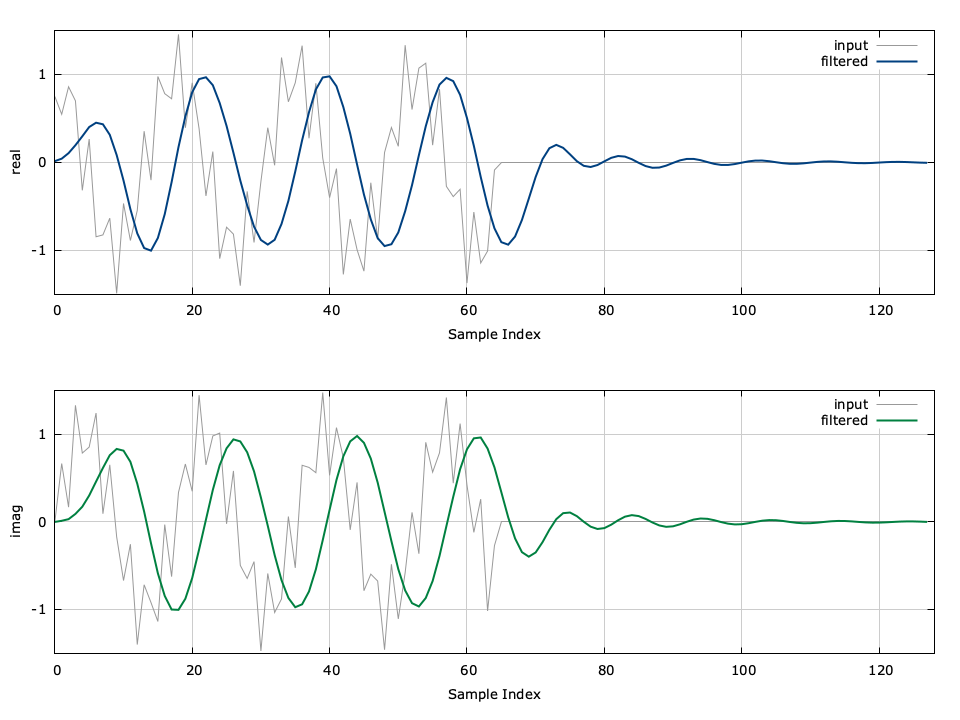# Infinite Impulse Response Filter (iirfilt)

The iirfilt_crcf object and family implement the infinite impulse response (IIR) filters. Also known as recursive filters, IIR filters allow a portion of the output to be fed back into the input, thus creating an impulse response which is non-zero for an infinite amount of time. Formally, the output signal $$y[n]$$ may be written in terms of the input signal$$x[n]$$ as

$$y[n] = \frac{1}{a_0} \left( \sum_{j=0}^{n_b-1}{ b_j x[n-j] } - \sum_{k=1}^{n_a-1}{ a_k y[n-k] } \right)$$

where $$\vec{b} = [b_0,b_1,\ldots,b_{n_b-1}]^T$$ are the feed-forward parameters and $$\vec{a} = [a_0,a_1,\ldots,a_{n_a-1}]^T$$ are the feed-back parameters of length $$n_b$$ and $$n_a$$ , respectively. The $$z$$ -transform of the transfer function is therefore

$$H(z) = \frac{Y(z)}{X(z)} = \frac{\sum\limits_{j=0}^{n_b-1}{b_j z^{-j}}} {\sum\limits_{k=0}^{n_a-1}{a_k z^{-k}}} = \frac{ b_0 + b_1 z^{-1} + \cdots + b_{n_b-1} z^{n_b-1}} { a_0 + a_1 z^{-1} + \cdots + a_{n_a-1} z^{n_a-1}}$$

Typically the coefficients in $$H(z)$$ are normalized such that $$a_0=1$$ .

For larger order filters (even as small as $$n\approx 8$$ ) the filter can become unstable due to finite machine precision. It is often therefore useful to express $$H(z)$$ in terms of second-order sections. For a filter of order $$n$$ , these sections are denoted by the two$$(L+r)\times 3$$ matrices $$\vec{B}$$ and $$\vec{A}$$ where $$r=n \mod 2$$ (0 for odd $$n$$ , 1 for even $$n$$ ) and $$L=(n-r)/2$$ .

$$H_d(z) = \left[ \frac{B_{r,0} + B_{r,1}z^{-1}} {1 + A_{r,1}z^{-1}} \right]^r \prod_{k=1}^{L} {\left[ \frac{B_{k,0} + B_{k,1}z^{-1} + B_{k,2}z^{-2}} {1 + A_{k,1}z^{-1} + A_{k,2}z^{-2}} \right]}$$

Notice that $$H(z)$$ is now a series of cascaded second-order IIR filters. The sos form is practical when filters are designed from analog prototypes where the poles and zeros are known. liquid implements second-order sections efficiently with the internal iirfiltsos_crcf family of objects. For a cascaded second-order section IIR filter, use iirfilt_crcf_create_sos(B,A,n) . See also : iirdes (IIR filter design) in[ref:section-filter-iirdes] .

Listed below is the full interface to the iirfilt family of objects. The interface to the iirfilt object follows the convention of other liquid signal processing objects; while each method is listed for iirfilt_crcf , the same functionality applies to iirfilt_rrrf and iirfilt_cccf .

• iirfilt_crcf_create(*b,Nb,*a,Nb) creates a new iirfilt object with$$N_b$$ feed-forward coefficients $$\vec{b}$$ and$$N_a$$ feed-back coefficients $$\vec{a}$$ .
• iirfilt_crcf_create_sos(*B,*A,Nsos) creates a new iirfilt object using $$N_{sos}$$ second-order sections. The $$[N_{sos} \times 3]$$ feed-forward coefficient matrix is specified by $$\vec{B}$$ and the $$[N_{sos} \times 3]$$ feed-back coefficient matrix is specified by $$\vec{A}$$ .
• iirfilt_crcf_create_prototype(ftype,btype,format,order,fc,f0,Ap,As) creates a new IIR filter object using the prototype interface described in [ref:section-filter-iirdes-iirdes] . This is the simplest method for designing an IIR filter with Butterworth, Chebyshev-I, Chebyshev-II, elliptic/Cauer, or Bessel coefficients.
• iirfilt_crcf_destroy(q) destroys an iirfilt object, freeing all internally-allocated memory arrays and buffers.
• iirfilt_crcf_print(q) prints the internals of an iirfilt object.
• iirfilt_crcf_clear(q) clears the filter's internal state.
• iirfilt_crcf_execute(q,x,*y) executes one iteration of the filter with an input $$x$$ , storing the result in $$y$$ , and updating its internal state.
• iirfilt_crcf_get_length(q) returns the order of the filter.
• iirfilt_crcf_freqresponse(q,fc,*H) computes the complex response $$H$$ of the filter at the normalized frequency $$f_c$$ .
• iirfilt_crcf_groupdelay(q,fc) returns the group delay of the filter at the normalized frequency $$f_c$$ .

Listed below is a basic example of the interface. For more detailed and extensive examples, refer to examples/iirfilt_crcf_example.c in the main liquid project source directory.

#include <liquid/liquid.h>

int main() {
// options
unsigned int order=4;   // filter order

unsigned int n = order+1;
float b[n], a[n];

// ... initialize filter coefficients ...

// create filter object
iirfilt_crcf q = iirfilt_crcf_create(b,n,a,n);

float complex x;    // input sample
float complex y;    // output sample

// execute filter (repeat as necessary)
iirfilt_crcf_execute(q,x,&y);

// destroy filter object
iirfilt_crcf_destroy(q);
}Figure [fig-filter-iirfilt_crcf]. iirfilt_crcf (infinite impulse response filter) example.

An example of the iirfilt can be seen in[ref:fig-filter-iirfilt_crcf] in which a low-pass filter is applied to a signal to remove a high-frequency component.